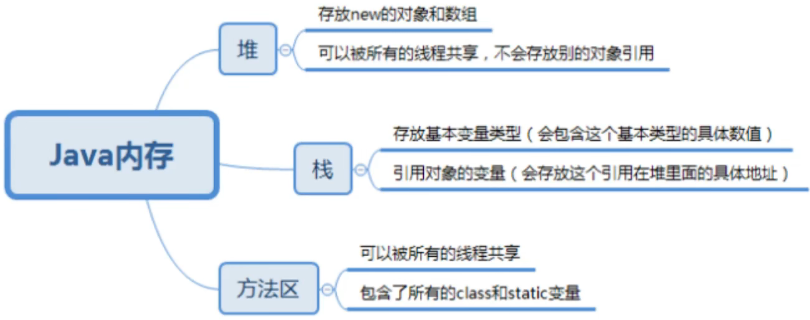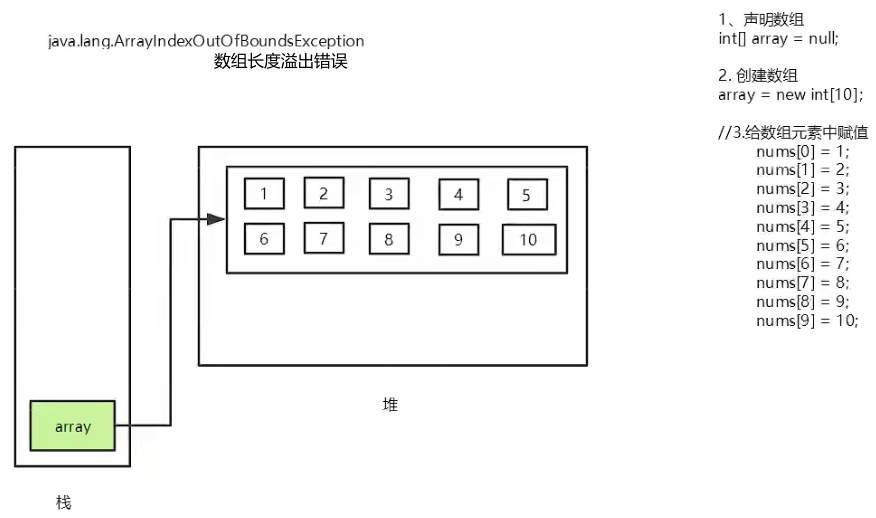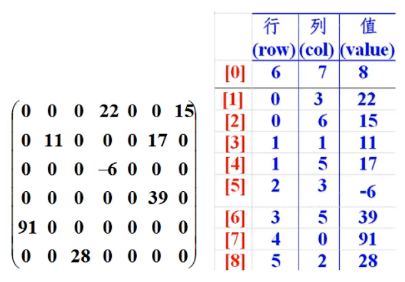﻿• 价格透明
• 信息保密
• 进度掌控
• 售后无忧# 【JAVA学习第四天（上）】

### 文章目录

• 前言
• 一、数组
• 1，数组的定义
• 2，数组的三种初始化
• 3，内存分析
• 4，数组的基本特点
• 5，Arrays类中常用的功能
• 6，冒泡排序
• 7，稀疏数组

# 前言

`提示：这里可以添加本文要记录的大概内容：`

`提示：以下是本篇文章正文内容，下面案例可供参考`

# 一、数组

### 1，数组的定义

``````声明数组（首选第一种）
1，dataType[] arrayRefVar;
int[] numbs;//声明一个数组
numbs = new int;//创建一个数组，可以存放10个int类型的数字
//给数组元素赋值
numbs = 1;
numbs = 2;
numbs = 3;
.........
numbs = 10
//若没有赋值，则为默认值。
2,dataType arrayRefVar[];

arrayRefVar.length  //获取数组长度
``````

### 2，数组的三种初始化

``````1，静态初始化
int[] a = {1,2,3};
int[][] a = {{1,2,3},{1,2,3}}//二维数组
2，动态初始化：包含默认初始化
int[] a = new int;//创建一个数组
a = 1;//为数组元素赋值
a = 2;
3，数组的默认初始化
数组是引用类型，它的元素相当于类的实例变量。
因此数组一经分配空间，其中的每个元素也被按照示例变量同样的方式被隐式初始化。
``````

### 3，内存分析### 4，数组的基本特点

a，长度在创建时确定，不可更改。
b，元素之间必须是相同类型。
c，元素类型可以是任意数据类型，包括基本类型和引用类型。
d，数组变量属引用类型，数组也可以看成是对象，数组中的每个元素相当于该对象的成员变量。

### 5，Arrays类中常用的功能

a，给数组赋值：通过fill方法，代码示例如下：

``````Arrays.fill(array,0);//array 为需要填充的数组，0为需要填充的值。
Arrays.fill(array,2,4,8);//2表示起始位置，4表示结束位置，为2到4之间的（2，3）元素赋值为8
``````

b，对数组排序：通过sort 方法，按升序排序，代码示例如下：

``````Arrays.sort(array);//把数组array 进行排序
System.out.pringln(array);//对排序完的数组进行输出
``````

c，比较数组：通过equals 方法比较数组中元素值是否相等。

``````Arrays.equals(array1, array4);//结果是布尔值
``````

d，查找数组元素：通过binarySearch 方法能对排序好的数组进行二分查找法操作

``````Arrays.binarySearch(array,0,5,3);//array为数组，0和5表示所取数组的下标段，3为目标
Arrays.binarySearch(array,3);//array为数组，3为目标

``````

### 6，冒泡排序

``````for (int i = 0; i < array.length-1; i++) {
for (int j = 0; j < array.length-1-i; j++) {
if (array[j+1] < array[j]){
temp = array[j];
array[j] = array[j+1];
array[j+1] = temp;
}
}
}
``````

### 7，稀疏数组``````public class ArrayNew {
//原数组
public static void main(String[] args) {
int[][] array = new int;
array = 2;
array = 5;
int sum = 0;

for (int i = 0; i < array.length; i++) {
for (int j = 0; j < array[i].length; j++) {
if(array[i][j] != 0){
sum++;
}
System.out.print(array[i][j]+"  ");
}
System.out.println("");
}
System.out.println("===============================================");
//创建稀疏数组
int[][] Array = new int[sum+1];
Array = array.length;
Array = array.length;
Array = sum;
int a = 1;
for (int i = 0; i < array.length; i++) {
for (int j = 0; j < array[i].length; j++) {
if(array[i][j] != 0){
Array[a] = i;
Array[a] = j;
Array[a] = array[i][j];
a++;
}
}
}
//输出稀疏数组
for (int[] each : Array) {
for (int i : each) {
System.out.print(i+"    ");
}
System.out.println();
}
System.out.println("===============================================");
//从稀疏数组变回原数组
int[][] arrayNew = new int[Array][Array];
for (int i = 1; i < Array.length; i++) {
arrayNew[Array[i]][Array[i]] = Array[i];
}
for (int[] ints : arrayNew) {
for (int anInt : ints) {
System.out.print(anInt + "   ");
}
System.out.println();
}
}
}
``````### 低价透明### 金牌服务### 信息保密